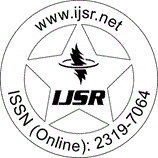International Journal of Science and Research (IJSR)
Call for Papers | Fully Refereed | Open Access | Double Blind Peer Reviewed

Research Paper | Statistics | Kenya | Volume 3 Issue 8, August 2014

# Jackknife Variance Estimation of Uniformly Minimum Variance Unbiased Estimates and the Maximum Likelihood Estimates for the Gamma Probability Density Function

Lillian A. Oluoch | Leo O. Odongo

Abstract: The gamma distribution is an important life time distribution. However, the drawback it faces in application is that the function useful in survival analysis and life testing is that its survivor function and hence hazard function does not exist in closed form. In this project we investigated the performance of the MLE and UMVUE of the gamma pdf. A simulation study of 100 runs was carried out in R- program by fixing the shape parameter at 0.5 and 1.0 for small (n=10), moderate (n=30) and large (n=50 and n=100) sample sizes. For each fixed, the shape parameter was varied at = 0.1, 0.5, 1.5, 2.0. Jackknife variance estimates for the MLEs and UMVUEs for the gamma pdf were obtained. Confidence intervals for the pdf were constructed and their coverage probabilities investigated at each nominal confidence coefficient. The results indicated that the Jackknife estimates of variance of the UMVUE are fairly stable compared to their MLE counterparts. In most cases the coverage probabilities of the UMVUE are generally closer to the nominal confidence coefficient than their MLE counterparts.

Keywords: uniform minimum variance unbiased estimates, Maximum likelihood estimates, Jackknife variance estimation, Gamma probability density function

Edition: Volume 3 Issue 8, August 2014,

Pages: 2081 - 2087